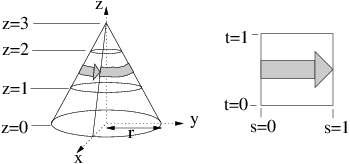UNB/ CS/ David Bremner/ teaching/ cs4735/ milestones/ CS4735 Assignment 5
• Due 2016-11-18, 17:30

### Part 1: Textures

1. Suppose you have a triangle with vertices `a=(a_x, a_y, a_z)`, `b=(b_x, b_y, b_z)` and `c=(c_x, c_y,c_z)` and a texture with coordinates `[0, 1] × [0, 1]`. Describe a transformation matrix that maps vertex a to texel `(0, 0)`, vertex b to texel `(0, 1)` and vertex `c` to texel `(1, 1)`.

2.We wish to wrap a rectangular texture around the central third of the cone in the figure. (Thus the bottom edge of the texture coincides with z = 1 and the top edge coincides with z = 2.) As s varies from 0 to 1, the texture should make one full revolution around the cone, starting from directly above the x axis. Give the inverse wrapping function, which maps a point (x, y, z) on the central third of the cone to texture coordinates (s, t).

3. Suppose we have a cube with vertices (0, 0, 0), (0, 0, 1), …(1, 1, 1) (in model coordinates). Give a mathematical function suitable to texture the faces of the cube into a k × k checkerboards (where k is a parameter of your function).

### Part 2: Interpolation

Consider a cube drawn using 12 triangles, 2 per face. Give examples illustrating different results from

1. Bilinear interpolation on quadrilaterals versus barycentric interpolation on triangles.

2. Perspective correct interpolation on triangles, versus interpolation in window coordinates. Assume a screen (near clipping plane) at distance 1.